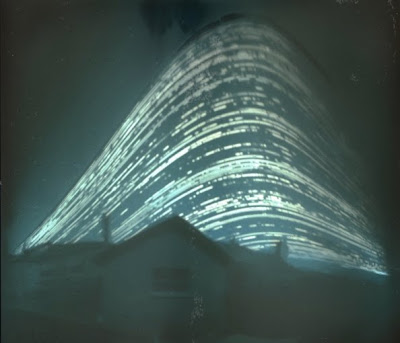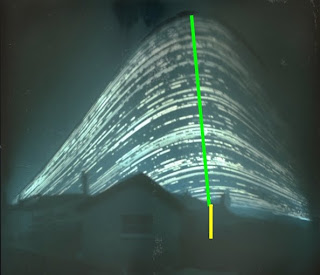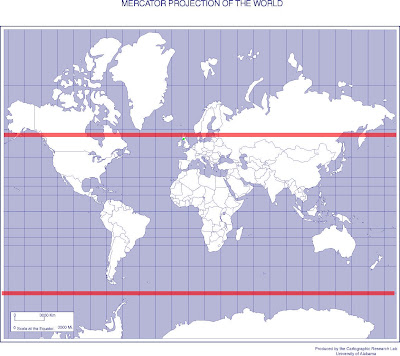# Locating the Sun Photo

This photo has been making the rounds on the internet lately:Originally from here. Its a time lapse photo of the sun taken from a pinhole camera from June until December. The real question is: Where was this photo taken?

#### Wandering Sun

Well, first lets think a second about why the sun would wander in the sky. As you may already know, the earth's pole is not aligned with our solar system's axis. The earth's axis has what is called a 23.5 degree axial tilt, or obliquity. As a result, if you sit at a fixed latitude on the earth, then as the earth revolves around the sun and the year goes by, the angle the sun makes with the horizon changes. We need to estimate how much the sun's angle in the sky changes. Consider the following diagramYou'll notice that the angle that the dashed line towards the sun makes with respect to the dotted horizon line, changes when the earth is on either side of the sun. But how much does it change? We can estimate it by realizing that the angle of that triangle centered at the sun is very small. Very small indeed. We could estimate its size by: $$\arctan \frac{R_{\text{Earth}}}{D_{\text{Earth-Sun}}} \approx 0.002^{\circ}$$ Very small. So, knowing that the one angle is very tiny, and remembering that the sum of all of the angles in a triangle must add up to 180 degrees, we can calculate how much that angle in the sky changes. Notice that the angle between the line pointing to our latitude of interest and the equator has a known angle, namely the angle there is our latitude +/- 23.5 degrees. So if that is the angle our observation point makes with the horizontal, by the reasoning above, the angle that the sun makes with respect to our observation point ought to be 180 - our latitude +/- 23.5 degrees. So on either side of the sun, the difference in this angle from summer to winter is just twice 23.5 degrees of 47 degrees. So, regardless of where you are on the earth, between when the sun is highest in the sky, and when it is lowest in the sky, there ought to be a 47 degree difference. (Assuming of course you can see the sun year round, which you can't if you live too far north). Great. So we know that the difference between the height of the traces in the picture and the bottom needs to be 47 degrees. But that still doesn't help us calculate the latitude the picture was taken at.

#### Finding Latitude in theory

Looking at our diagram again, we notice that when the sun is lowest in the sky, we can calculate directly what angle the sun should make with respect to the horizon (psi) (We already obtained the upper angle in our triangle, the last part is realizing that the angle to the horizon from the radial line is just 90 degrees). We obtain $$\psi = \theta - 90^\circ + 23.5^\circ = \theta + 66.5^\circ$$ So, turning it on its head, we can calculate the latitude (theta) we are at if we know the lowest angle the sun makes in the sky $$\theta = 66.5^\circ - \psi$$ Great.

#### Finding Latitude in Practice

So, now I know two things. The total angle difference between the upper most the sun gets and the lowest point in the sky is 47 degrees. And I also know that if I know the angle between the horizon and the lowest point the sun gets in the sky, I can calculate the latitude. So how am I going to do this, well, I just took the picture and opened it up in Gimp, and used the measure tool to get the difference between the highest and lowest points in the sky in pixels, and the distance to the horizon in pixels. Here's one example of my guesses:Getting the differences in height for the sun was relatively straight forward. In order to get the distance to the horizon, I had to guess a little bit. I just lined it up by eye to where I thought the horizon should be in the picture since its not visible. Why does this do me any good? Well assuming the picture doesn't have too much distortion, since I know the one angle is 47 degrees, I can make a comparison between the pixel lengths. Lets say in the picture above I call the length of the green line B, and the length of the yellow line S, then I can estimate $$\frac{\psi}{S} = \frac{47^\circ}{B}$$ which gives me a measure on psi. Since I was doing this by eye, I repeated the measurement ten times independently for each, obtaining in the end $$B = 356 \pm 2 \qquad S = 66 \pm 6$$ And I have a complete formula for my estimation of the latitude $$\theta = 66.5^\circ - 47^\circ \frac{S}{B}$$

#### Results

This allowed me to estimate the latitude at 58 +/- 1 degree latitude. Naturally, I can't tell the difference between 58 degrees north or 58 degrees south. To get a better idea of what this guess looks like, here I've overlayed the possible locations in greenYou'll notice that there isn't much life down at 58 degrees south of the equator. So, I reckon the picture was taken in the northern hemisphere. Surfing around on the webpage where the picture was found, you'll first notice that its taken from a co.uk site (Great Britain), and if you go to the homepage, Mr. Mallon has a weather widget which says Bellshill. Google Maps tells me that Bellshill, UK is at about 56 degrees North. I've tried to put a green dot on the map above near where Bellshill actually is (its hard to see). Not bad for a night's work.